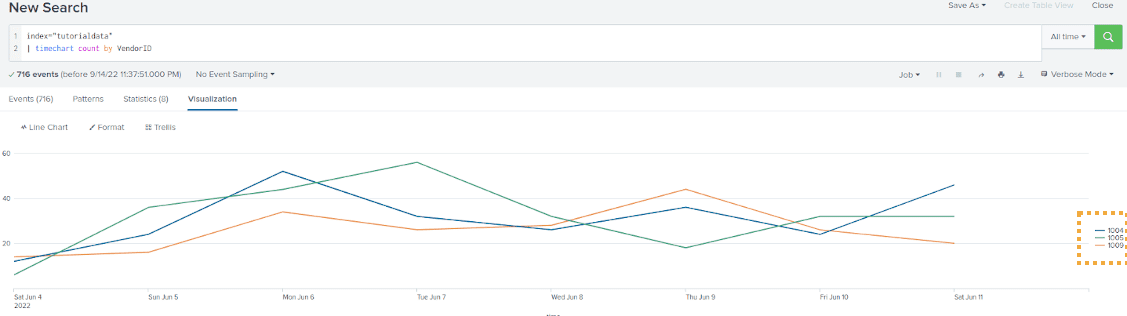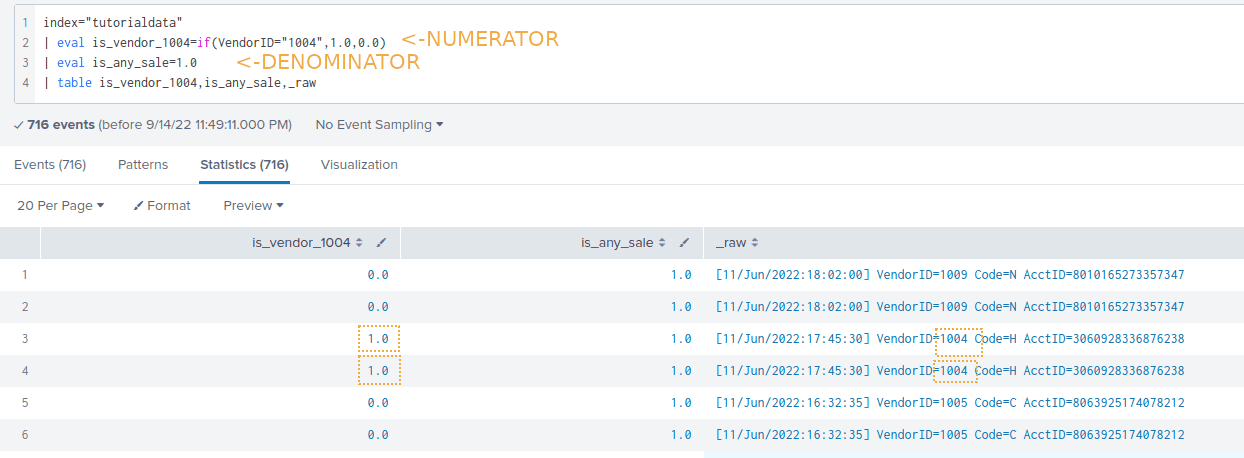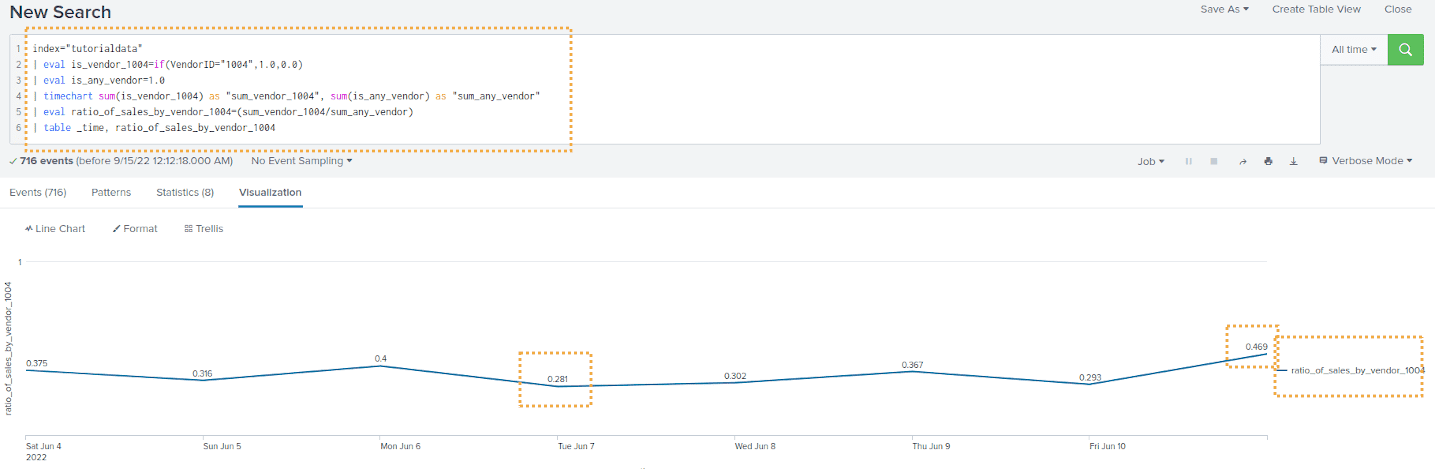Splunk Examples: Combining Streams of Events

# Splunk Examples: Combining Streams of Events

Last updated:

All examples use the tutorial data from Splunk running on a local Splunk version

## Calculate ratio between two events

Useful to chart timeout rates, failure rates, etc

Say you have log the number of sales by each VendorID, per unit time):

index="tutorialdata"
| timechart count by VendorIDThree events taking place

Ỳou now want to plot the ratio between VendorID 1004 and the total, to see what fraction of the total sales was made by 1004:Create one variable for the numerator and another one for the denominator like this

Final query: Plot the ratio of sales made by Vendor 1004 over time:

Note that we call timechart and then end with a call to table:

index="tutorialdata"
| eval is_vendor_1004=if(VendorID="1004",1.0,0.0)
| eval is_any_vendor=1.0
| timechart sum(is_vendor_1004) as "sum_vendor_1004", sum(is_any_vendor) as "sum_any_vendor"
| eval ratio_of_sales_by_vendor_1004=(sum_vendor_1004/sum_any_vendor)
| table _time, ratio_of_sales_by_vendor_1004The rate of sales made by Vendor 1004 ranges
from 0.281 to 0.469, depending on the day!